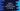# Python program to calculate total vowels in a string## Total vowels in a string using Python :

In this python programming tutorial, we will learn how to calculate the total number of vowels in a string.

The user will provide the string. Our program will calculate the number of vowels and print out the result.

## Algorithm to use :

We will use the following algorithm to solve this program :

1. Take the string from the user and store it in a variable.
2. Initialize one variable to 0. This variable will be used to store the count of vowels in the string.
3. Traverse through the characters of the string using a for loop.
4. On each iteration of the loop, check if the current character is a vowel or not.
5. If the current character is a vowel, increment the count variable by 1.
6. Finally, print out the vowel count.

### Python program :

``````#1
def isVowel(c):
return (c == 'a' or c == 'e' or c == 'i' or c == 'o' or c == 'u' or c == 'A' or c == 'E' or c == 'I' or c == 'O' or c == 'U')

#2
input_str = input("Enter the string : ")
vowel_count = 0

#3
for ch in input_str:
#4
if isVowel(ch):
vowel_count += 1

#5
print("Total vowel count : {}".format(vowel_count))``````

This program is also available here in Github.

### Explanation :

The commented numbers in the above program denote the step numbers below :

1. isVowel method is used to check if a character is a vowel or not. It takes one character as a parameter and compares it with all upper-case and lower-case vowels. If it is a vowel, it returns True, else it returns False.
2. Ask the user to enter a string. Read the string and store it in the inputstr_ variable. Initialize variable vowelcount_ to 0. This variable will hold the_ total count of vowels_ in the user input string.
3. Using one for loop, iterate through each character of the string.
4. For each character, check if it is a vowel or not. If it is a vowel, increment the variable vowelcount_ by 1.
5. Finally, print out the total count of vowels vowelcount_

### Sample Output :

``````Enter the string : hello world
Total vowel count : 3

Enter the string : aeiou
Total vowel count : 5

Enter the string : aeiouAEIOU
Total vowel count : 10

Enter the string : 12345
Total vowel count : 0

Enter the string : This is a testing line
Total vowel count : 7

Enter the string : abcdefg
Total vowel count : 2``````

### Conclusion :

In this tutorial, we have learned how to iterate over the characters in a string in python and to find out the total number of vowels. Try to run the above example and drop one comment below if you have any queries.